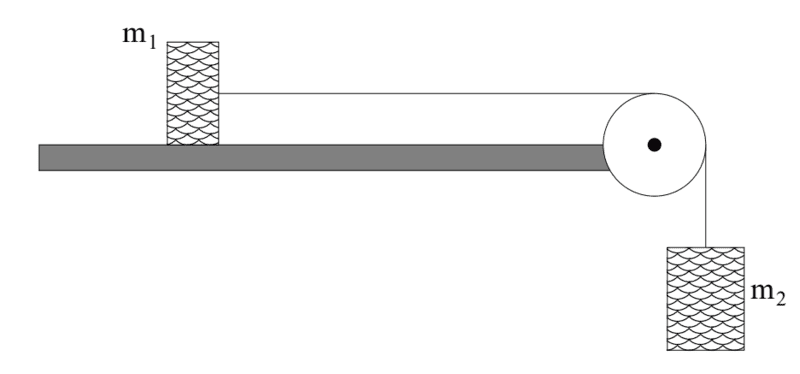# Two blocks, a fixed pulley and friction

SakuRERE

## Homework Statement

a mass m1 is attached to a second mass m2 by an acme (massless, unstretchable) string. m1 sits on a table with which it has coefficients of static and dynamic friction μs and μk respectively. m2 is hanging over the ends of a table, suspended by the taut string from an acme pulley. at time t=0 both masses are released.
1. what is the minimum mass m2,min such that the two masses begin to move?
2. If m2= 2m2,min, determine how fast the two blocks are moving when mass m2 has fallen a height H (assuming the m1 hasn't yet hit the pulley)?

Σf=ma
fsmax=μs*fn
vf2=vi2+2aΔx[/B]

## The Attempt at a Solution

for a:
I am not sure about how I understand the question, but what comes to my mind when reading ( Begin to move) is that still the system acceleration is zero and that they are asking for the Fsmax (the maximum static friction) just before the system starts to move and the friction becomes Kinetic friction of Fk.
so:
for block m1
Σƒ= m*a=0
fsmax=T
μs*Fn= T
μs*m1g=T
now for block m2:
Σƒ=m*a=0
m2g=T

and by this m2g=μs*m1g so m2=(μs*m1g)/g

section b)
I guess since the mass of m2 is doubled, that here we will deal with the kinetic friction rather than the static friction.
since both are linked by a rope with the same pulley than they must have the same acceleration, and we should find it.
and after finding it then we will use this equation:
vf2=vi2+2aΔx
where vi= 0
so vf=√(2aΔx).
am I right??#### Attachments

Homework Helper
Gold Member
so m2=(μs*m1g)/g
Yes, but you can simplify a little.
section b)
I guess since the mass of m2 is doubled, that here we will deal with the kinetic friction rather than the static friction.
since both are linked by a rope with the same pulley than they must have the same acceleration, and we should find it.
and after finding it then we will use this equation:
vf2=vi2+2aΔx
where vi= 0
so vf=√(2aΔx).
am I right??
Yes.

•SakuRERE
SakuRERE
but what is a
yes, of course, I will be substituting a in term of the given variables but I just didn't because I wasn't sure from my solution for section a at the first place. but now after your response, I will continue the solution. and regarding the H and Δx, I will be substituting Δx with H simply, but I wanted to write the equation in its original form so that everyone easily identifies it.
I will be happy if you grant me another help and answered this question:
for a moment I wondered, will the final velocity be the same for both blocks, I know they have the same acceleration and H will be the same as well but doesn't the friction affect the velocity of that block in the incline?

•